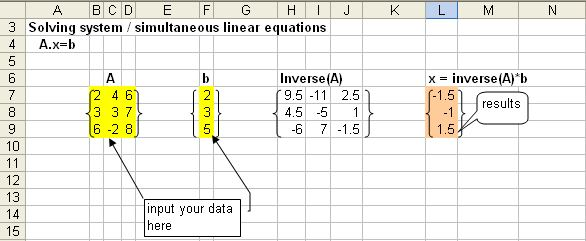## Solving Linear Equation using Excel

This tutorial will describe how to use an inverse function for matrix in Microsoft Excel to solve non-homogeneous simultaneous linear equations.

Suppose we want to solve simultaneous equations belowWe can convert the simultaneous equations above into matrix form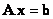where,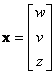and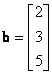The solution of the simultaneous equations can be easily obtained using inverse matrix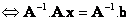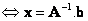Here is systematically how to do it in Microsoft Excel.

1. Input the numbers of matrix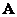and vector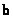2. Tell MS Excel the name of this matrix by menu Insert-Name-Define .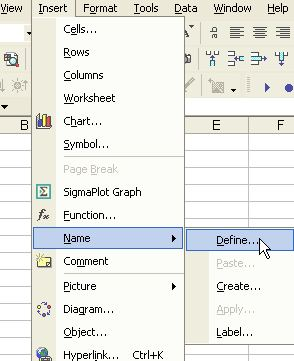3. A defined-name dialog will appear and type the name of matrixand refer to the range of the matrix, then click OK. In a similar way, you define the name of vector.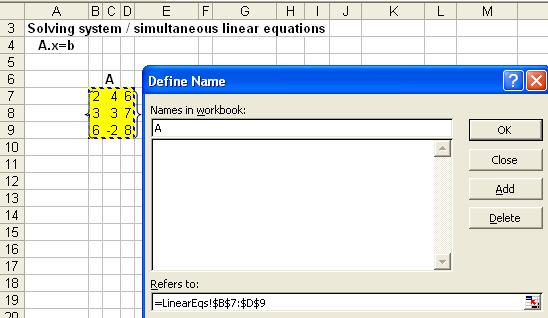4. In a cell where do you want to put the inverse of matrix, you type =MINVERSE() . It will give you only one number. Now using the mouse highlight the range where do you want to put the inverse of matrix. Because you know that the size of inverseis also 3 by 3 matrix, highlight only that range.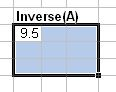While highlighting, press F2 and then Shift-Ctrl-Enter. You will get the inverse of matrix.